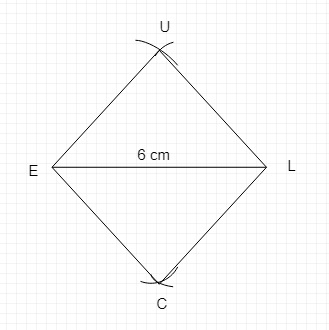# Construct a rhombus CLUE in which $CL = 7.5$ cm and $LE = 6$ cm.

Given :

$CL = 7.5$ cm and $LE = 6$ cm.

To do :

We have to construct a rhombus CLUE.

Solution :

We know that,

All the sides of a rhombus are equal.

Therefore,

$CL = LU = UE = EC = 7.5 cm$Steps of construction :

1. Draw a line $LE = 6$ cm.

2. Take a radius of 7.5 cm and draw two arcs on both sides of line LE, by taking

center L.

3. Take a radius of 7.5 cm and draw two arcs on both the sides of line LE, by taking the center E  such that both the arcs meet at C and U.

4. Now join CL, LU, UE, and EL.

CLUE is the required rhombus of side length 7.5 cm.

Updated on: 10-Oct-2022

30 Views